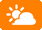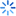|23 ~ 36℃ 多云 北京天气详情

 入住日期： 离店日期：酒店设施

• 商场
• 理发美容中心
• 残疾人客房
• 收费停车场
• 前台贵重物品保险柜
• 免费旅游交通图(可赠送)
• 自动取款机
• 咖啡厅
• 中餐厅
• 西餐厅
• 大堂吧
• 中央空调
• 电梯
• 大堂免费报纸
• 吸烟区
• 餐厅
• 空调
• 无烟楼层
• 多功能厅
• 公共区域闭路电视监控系统
• 公共音响系统
• 礼品廊
• 专用展览厅

• 叫车服务
• 会议厅
• 旅游票务服务
• 洗衣服务
• 商务中心
• 外币兑换服务
• 邮政服务
• 专职行李员
• 行李寄存
• 叫醒服务
• 送餐服务
• 24小时前台服务
• 租车服务
• 干洗
• 熨衣服务
• 礼宾服务
• 传真/复印
• 24小时大堂经理
• 商务服务
• 婚宴服务
• 信用卡结算服务
• 外送洗衣服务
• 专职门童
• 旅游票务专柜

• 茶室
• 按摩室
• KTV
• 舞厅

• 国内长途电话
• 国际长途电话
• 浴室化妆放大镜
• 24小时热水
• 免费洗漱用品(6样以上)
• 吹风机
• 拖鞋
• 书桌
• 多种规格电源插座
• 房内保险箱
• 电热水壶
• 咖啡壶/茶壶
• 免费瓶装水
• 迷你吧
• 小冰箱
• 淋浴
• 熨衣设备
• 雨伞
• 浴缸
• 独立淋浴间
• 中央空调
• 免费洗漱用品(6样以内)
• 唤醒服务
• 城景
• 花园景
• 享有风景
• 阳台
• 按摩浴缸
• 洗浴间电话
• 浴室
• 液晶电视机
• 衣柜/衣橱
• 针线包
• 遮光窗帘
• 手动窗帘
• 电子秤
• 卫星频道
• 电视机
• 闹钟
• 坐卧两用长沙发
• 特长睡床(超过两米)
• 床具:鸭绒被
• 床具:毯子或被子
• 地标景
• 沙发
• 电脑
• 备用床具
• 露台
• 有线频道
• 收费电影
• 厨房
• 房间内高速上网
• 电话
• 客房WIFI覆盖

可接受信用卡类型